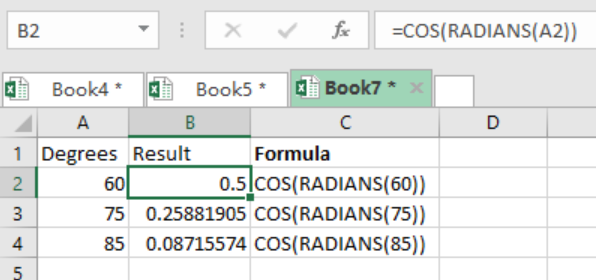Get instant live expert help with Excel or Google Sheets“My Excelchat expert helped me in less than 20 minutes, saving me what would have been 5 hours of work!”

#### Post your problem and you’ll get Expert help in seconds.

Your message must be at least 40 characters
Our professional Expert are available now. Your privacy is guaranteed.

# How to Use the COS Function in Excel

We can use the Excel COS function when looking for a cosine of an angle in radians. We can supply an angle to COS in degrees by simply multiplying the angle by PI()/180. To get it in radians, we can simply use the RADIANS function in excel. This post provides a summary of how to use the COS function in excel.Figure 1: How to use the COS function in Excel

## General syntax of the formula

`=COS(number)`

Where;

• Number- refers to the angle for which we want to find the cosine, the angle should be in radians.

## Understanding the formula

• The COS function only accepts angles in radians if it is to return the correct answer.
• For us to supply the angle in specified degrees, we have to multiply the angle by PI()/180.
• We can also use the RADIANS function in order to convert the angle into radians.

In the example above, we have the cosine of the 60 degrees as 0.5 as shown in cell B2. The formula of how we have arrived at the results in the example are shown in column C.

## Instant Connection to an Expert through our Excelchat Service

Most of the time, the problem you will need to solve will be more complex than a simple application of a formula or function. If you want to save hours of research and frustration, try our live Excelchat service! Our Excel Experts are available 24/7 to answer any Excel question you may have. We guarantee a connection within 30 seconds and a customized solution within 20 minutes.

Solution examplesIf I merged a multi-row, multi-column selection. Iand types some text into the merged cell and then unmerges it, what will happen to the text?
Solved by S. Y. in 60 mins